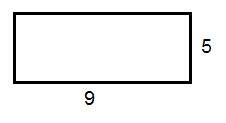# Calculating the area and the perimeter

The perimeter is the length of the outline of a shape. To find the perimeter of a rectangle or square you have to add the lengths of all the four sides. x is in this case the length of the rectangle while y is the width of the rectangle.

The perimeter, P, is:

$P= x+x+y+y$

$P=2x+2y$

$P=2(x+y)$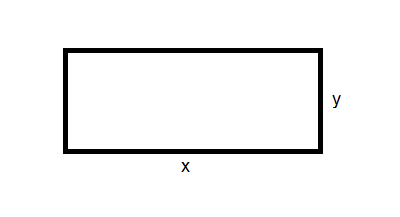Example

Find the perimeter of this rectangle: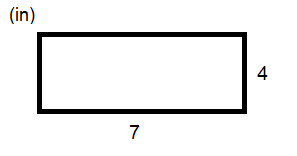$P=7+7+4+4$

$P=2\cdot 7+2\cdot 4$

$P=2\cdot (7+4)$

$P=2\cdot 11$

$P=22\,in$

The area is measurement of the surface of a shape. To find the area of a rectangle or a square you need to multiply the length and the width of a rectangle or a square.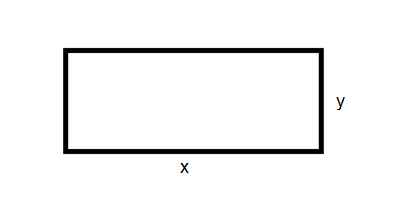Area, A, is x times y.

$A=x\cdot y$

Example

Find the area of this square.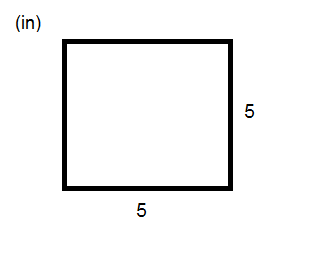$A=x\cdot y$

$A=5\cdot 5$

$A=25\,in^{2}$

There are different units for perimeter and area The perimeter has the same units as the length of the sides of rectangle or square whereas the area's unit is squared.

## Video lesson

Calculate the perimeter and the area in feet